By Ronnie Brown, Emeritus Professor, Bangor University, FLSW.

Abstract:

 The title refers to mathematics as a different way of looking at things, and as a new kind of mathematics in which symbols are no longer confined to a line, or even a page. To explain why we should want his freedom, we use squares, knots, string and computer graphics to illustrate some principal themes in mathematics: symmetry, motion, space. Finally, we try to catch a glimpse of the future. Talk given in 1992 as a Royal Institution Friday Evening Discourse, and to the British Association for the Avancement of Science as the Presidential Address to the Mathematics section, in Southampton. It is addressed to a general audience. Original version: Royal Institution Proceedings, Volume 64 pp207-243. Revised web version (colour graphics and some additions/corrections/clarifications to text) as pdf/html 2006 by Marcus Brown.

Contents. (The paper is split into 3 web pages for browsing. Select the full paper -PDF/HTML - for downloading/printing.)

 This page 1This web version of the paper includes images from the popularisation web site (click on the image) and accompanying CD. Contact the author for more information and to obtain a copy of the CD. For mathematicians: "From groups to groupoids: a brief survey" (ref ) . BOOK: Topology and Groupoids. R. Brown Book Surge LLC, 2006. Order from amazon.com. 2 4 Next page 7 9 Last page. 15 18 22 IX.Implications. References. 24 PDF.PDF version 24pp, 700KB OPEN/DOWNLOAD.

I. Introduction

Mathematics is an unseen, and usually unsung, part of our daily lives. It lies behind: econometric models; body scanners; aeroplane design; medical statistics; CD players; engineering; the theory which enabled the error correction to reconstruct stunning pictures from the Voyager II mission; the design of computers; and a host of others. Consider also the graphs we see in our daily papers: inflation against time; sales against time; risk of cancer against number of cigarettes per day. This concept of a graph derives from the application of algebra to geometry, through the Cartesian coordinates invented in the 17th century. So mathematics is involved in matters of life and death, profit and loss, work and play. Parts of the language of mathematics, for example, number and graph, devolved over the centuries into everyday life.

Mathematics also leads science. The great physicist, Paul Dirac, in one of his last addresses, explained his own credo (quoted in  p.63):

"One should allow oneself to be led in the direction which the mathematics suggests... one must follow up a mathematical idea and see what its consequences are, even though one gets led to a domain which is completely foreign to what one started with.... Mathematics can lead us in a direction we would not take if we only followed up physical ideas by themselves."

For mathematics to lead in this way, it has to exist. It is not generally recognised what an extraordinary range of new mathematics is being found. The aim of this Discourse is to describe just one example of new mathematics, which I and others like to call Higher Dimensional Algebra. It is a pleasure to convey to this meeting of the Royal Institution something of the story of what seems likely to be a new discipline in mathematics, and one which opens out a new world of concepts and applications.

I will explain also the background to this approach, not exactly with the original ideas, which are almost lost in the murk of history, but in a more concrete and explicit form, and so give some impression of how concepts can be brought out of the dark.

Let me stake my claim that mathematics is about ideas, concepts, and visions. The craft is how to make these real and rigorous.

II. Formulae in a Line or in a Plane

Here is an illustration of our idea of higher dimensional algebra. Consider a simple and well known formula,

(x - y)(x + y) = x2 - y2

but now with each symbol written on a children's block, as in Fig 1: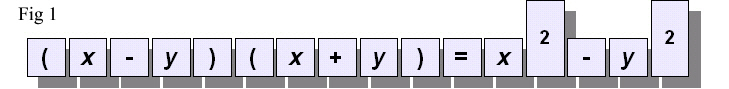If the formula is not familiar, that does not matter. Everyone knows mathematics is concerned with x 's and y 's and that formulae of this kind occur. But note one of the characteristics of this formula: each symbol in the formula is related to those to the left and those to the right, according to standard rules. Further, this arrangement corresponds to our standard mode of writing.A temptation with a row of children's blocks is to build them into a wall, as in Fig. 2. This unfortunately turns the formula into nonsense. It would be even worse if we built a tower!

However, this very temptation leads to some naive questions (and we know from history that the best science starts from simpleminded questions):

Is there some mathematics which corresponds to this new arrangement? Is the convention of mathematics on a line a necessity, or a question of habit? Suppose there were a mathematics, an algebra, in which symbols were related not only on a line, but also out of line, in a plane, up and down, or even out of the plane. What would this mathematics be like, and what would it do for us?

I shall immediately give an illustration of how thinking "out of line" ie in more dimensions, can be illuminating and suggestive. Consider the formula and the graphical representation in fig 3.
 2 × (2+3) = 2 × 2 + 2 × 3Fig 3

The arithmetical formula is correctly formed and perfectly straightforward to anyone with a knowledge of the arithmetical symbols and "in a line" structure. But without this mathematical language knowledge? The pictorial representation in 2 dimensions however can be regarded as self explanatory! In mathematics we want the notation to have a structure that aids intuition and calculation, to make things easier. Historically this kind of advance was obtained, for example, in moving from Latin (I, II, III IV etc) to Arabic numerals.

This questioning of our standard notation is the main background to this Address.

I would also like to give the authority of Einstein (1916) for the questioning of standard usage:

"For when I turn to science not for some superficial reason .... then the following questions must burningly interest me as a disciple of science: What goal will be reached by the science to which I am dedicating myself? To what extent are its general results `true'? What is essential and what is based only on the accidents of development?...[my italics] ...It is therefore not just an idle game to exercise our ability to analyse familiar concepts, and to demonstrate the conditions on which their justification and usefulness depend, and the way in which these developed, little by little..."

The question on the rearrangement of the blocks is actually more complicated than necessary, since the formula given includes addition, brackets, multiplication, and equals. Here is a simpler question. It is standard practice in mathematics to express a diagram of labelled arrows as in Fig. 3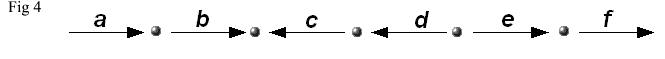by the formula

abc-1d-1ef

where a reversed arrow is represented by the inverse, for example by c-1 instead of c. This presents no problem at all, and is very familiar. Now consider the following diagrams (Fig. 4, Fig 5, Fig 6):Fig 5. Note that each edge has an arrow and each square is labelled. Question: Is there an algebra to describe the diagram: to express mathematically that the big square is made up of all the little squares? In Fig 6 we have labelled the edges of one of these small squares.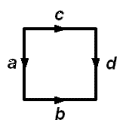Fig 6 We can easily write down precisely and mathematically some basic algebraic questions: what it means to say "going round one way is the same as going round the other"  in symbols : ab = cd ; or we can say the top edge is a combination of the others: c = abd-1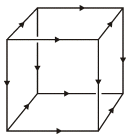Fig 7. We move up one dimension, from a square to a cube. The cubes' edges are given arrows in a consistent way, and one should imagine that the faces are labelled. Question: Is there a meaning to: going round one way is the same as going round the other? the top face is a combination of the other faces? And how might we represent these algebraically?

What is embarrassing is that, while the one-dimensional answers are simple and straightforward, with formulae on a line of a standard type, yet it is not apparent where to start to write down anything which makes sense in answer to the question in just one higher dimension.

These questions occurred to me in 1966. I had an idea that there was a mathematics in which this 2-dimensional algebra made sense, and which would yield a formulation and proof of a new and potentially important version of some basic ideas in topology, centred round how to model the building up of big spaces from their parts. This application (what are called the Van Kampen Theorems) is still for me the core of the future of higher dimensional algebra. But note the interesting point of methodology: I was not trying to solve a specific problem, of the form "Is statement A true or false?", but instead proposing to produce a new type of mathematics, with certain characteristics, and which, if realised, should allow for the solution of problems, without, at that early stage, specifying which problems.

The process here is that of devising a new language to express and realise intuitions. It involves the formulation of new concepts, and this leads to new problems. This is one of the major forms of progress in Mathematics, allowing the opening up of new arenas.

The mathematician regarded as the the 20th century master of the development of new concepts referred, in correspondence to this author, to

"the difficulty of bringing new concepts out of the dark"; (A. Grothiendick pers comm.).

How new concepts and ideas can change things is entirely topical of course in this era of the internet and rapid technological development. A good example from mathematics is the concept of zero, which we understand became accepted (in western Europe) as part of the renaissance and is a foundation stone of all subsequent arithmetical calculation. Indeed what mathematics does is develop language for expression, description, verification and calculation.

It was a surprise that just to make initial progress was not easy, but the intuitions were so strong the ideas would not go away. The programme turned into a major undertaking, to date involving, with me alone, eleven co-authors from seven countries (Hong Kong, UK, Germany, France, Poland, Ireland, USA), and ten research students at Bangor and Durham (who made crucial contributions to the theory). The first paper was published in 1976, ten years after the start. By now there is a rich and tightly knit theory which encapsulates the original intuitions, performs the job that was intended, allows for new calculations, and opens out many possibilities for the future.

The first question was where to start. The aim was this potential Generalised Van Kampen Theorem. In its standard form in dimension 1, this theorem used the notion of abstract group, and I had shown in 1965 that the standard theorem could be improved by using the less usual notion of groupoid instead of group. It seemed reasonable, even necessary, to ask to what extent groupoids, rather than groups, could be used in the higher dimensional theory. It seemed no one else had asked this question, partly because groupoids were generally not well regarded as a tool in mathematics.

To show why these questions had prospects of being important, I will explain the relevance of the terms group and groupoid to three great themes in mathematics: Symmetry, Space and Motion. I will also use these themes to describe the main features of the search for the unknown forms of higher dimensional algebra.

III. Symmetry and Abstract Groups

The mathematical structure expressing the notion of symmetry is that of abstract group. To explain this, we examine the nature of symmetry.

The importance of symmetry

Here is what the great mathematician, Hermann Weyl, wrote on symmetry:

"Symmetry establishes a ridiculous and wonderful cousinship between objects, phenomena and theories outwardly unrelated: terrestrial magnetism, woman's veils, polarized light, natural selection, the theory of groups, invariants and transformations, the work habits of bees in the hive, the structure of space, vase designs, quantum physics, scarabs, flower petals, X-ray interference patterns, cell division in sea urchins, equilibrium positions of crystals, Romanesque cathedrals, snowflakes, music, the theory of relativity."

That is quite a list - and only a sample!

To see how mathematics comes in, and allows calculations, let us take a simple example:

Symmetry of a square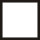We represent the symmetry of the square by considering all the eight movements which take the square to itself. This consideration of all movements, this global viewpoint, is a common feature of mathematical practice. Among all these movements, we consider two basic ones from which all the other symmetries of the square can be built:

x =: Rotation anticlockwise through 90
y =: Reflection in a vertical line

So x is represented as in Fig. 8 and y is represented as in Fig. 9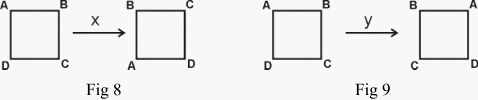Here x and y move whatever is in the positions at the corners into new positions. Given a symmetry operation, we can do one after the other. If we do x , the rotation through 90° , four times, we get back to where we started (Fig. 10)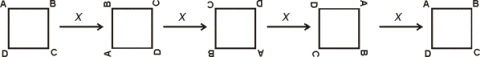Fig 10

If we do y twice, we also get back to where we started. These facts are expressed symbolically by:
 x4 = 1, y2 = 1.

Notice that we use the symbol 1 to denote the operation of leaving the square alone, of doing nothing. These rules are called relations on x and on y.

A third rule or relation is more surprising, and cumbersome to express in words: It can be shown as in Fig 11:
 xyxy = 1.Fig 11

It is now the technical job of a mathematician to work within these rules, and to make deductions. For example, we can find that
 x-1 = x3, y-1 = y, xy = yx3, yx = x3 y .

These last two rules can be summarised as: x changes to x3 when it moves past y.

This fact, and the rules x4 = 1, y2 = 1, are all that is necessary to allow for calculations in this group of symmetry operations of a square. The rule xy = yx3 is surprising, since multiplication of ordinary numbers satisfies the rule : xy = yx . This rule is called the COMMUTATIVE law.

That it does not hold for symmetries in general is crucial for the theory of symmetry, and will recur when we come to discuss the higher dimensional theory.

The eight symmetry operations of a square, with their 64 products, form an example of a symmetry group. This symmetry group is a special case of the general notion of abstract group.

Abstract group and structure.

Here we come to a key notion we have to explain, the notion of an abstract structure. It is a very difficult notion, but it is at the heart of the nature of mathematics. These structures are among the objects of mathematics in the same way as animals are the subject of zoology, molecules of chemistry, and so on. What then are structures?

Mathematical structures are used to describe not things themselves, but relations between things, or how parts of objects are related. This involvement with relations between things is why mathematics is an abstraction from the real world. This also is its power, since the patterns of relations we perceive and abstract can often be found to occur in a variety of places and situations, and this leads to analogies and transfers of information.

A mathematical structure can be thought of as like a game, consisting of pieces, modes of combination, and rules (although a precise definition requires quite sophisticated mathematical language). Once you accept a game, then you work out the consequences of the rules, and see what the game is like, and what will happen as the players pursue various actions. A similar procedure applies to mathematical structures.

This working out of the consequences of the rules is not a mechanical procedure. E Wigner writes in his famous essay "The unreasonable effectiveness of mathematics in the physical sciences": "I would say that mathematics is the science of skillful operations with concepts and rules invented just for this purpose [i.e. of skillful operation with concepts and rules!]. ...the principal emphasis is on the invention of concepts."

Wigner also emphasises the need to define concepts beyond those contained in the axioms, the basic rules, and "with a view to permitting ingenious logical operations which appeal to our aesthetic sense both as operations and also in their results of great generality and simplicity". 

Now I come to the particular mathematical structure of an abstract group. You notice that I considered all the eight symmetry operations of a square, and then considered doing first one and then another. It is this combination (also called product, or composition) that is crucial and for which we try to lay down laws or rules, and so form a mathematical structure. The laws for this structure have to be sufficiently general to be of wider implication than just the one example with which we are dealing, but also sufficiently special to include it.

It is no simple matter to formulate useful mathematical structures. They tend to evolve over years, decades, or centuries, as mathematicians find the rules and combinations that are commonly used, and see what is the pattern that keeps on recurring, and must be given for convenience a special name and habitation.

For our concept of an abstract group, we start with a collection of things, which might be anything, from numbers, to movements of a square or of a triangle, but there has also to be given a method of combining any two of our things, say x and y , to get a third, written say xy . We have to consider both the things and their method of combination together, and we have to suppose satisfied three laws, which are called the axioms for an abstract group.

Axioms of an Abstract Group.

1. There is an identity , usually written 1, so that for any x we have 1 × x = x × 1 = x .
2.  Any x has an inverse x -1 for which xx -1 = x -1x = 1.
3.  Associativity , which essentially states that there is only one way of multiplying three elements one after the other, in a specific sequence. For those familiar with symbols, this says that x (yz ) = (xy )z .

For numbers and multiplication, for example, with x = 3, y = 5, z = 7, this rule reads 3 × 35 = 15 × 7 . This notion of abstract group, as above, has been found over the last 200 years to be one of the of the central concepts of mathematics. The formulation of this concept involved the work of many mathematicians and scientists, from ancient Greece, through to the middle of the nineteenth century.

The apparent simplicity of the axioms for an abstract group disguises their power. Groups are used in chemistry, crystallography, the physics of fundamental particles, the design of wave guides, dynamical systems, in fact wherever there is a notion of symmetry, or of some kind of repeated, reversible motion.

Note that in the axioms for a group, we single out the properties of the identity element, namely the symmetry which leaves things alone. Without this "trivial" identity element of symmetry, we cannot formulate the notion of group! This illustrates a feature of mathematics: the importance of pedantry, or of noticing everything that happens, or attention to detail. I have already referred to the importance of `zero' in the introduction. Zero was used in India in the 2nd century BC, and made its way to Europe in the 15th century AD, as a part of the Arabic system of numerals. This system led to the invention of double entry book-keeping, and so, it is believed, to the prosperity of Venice. All this arises from counting the number of objects in an empty box, an apparently foolish undertaking! We will see more of identity operations later.

The symmetry group of the square has 8 elements. It is a fairly simple structure. The symmetry group of a cube has 48 elements. There is an enormous variety of groups. The classification of finite groups, and particularly what are called, possibly misleadingly, simple groups, has been an astonishing undertaking, involving many mathematicians, and the final proof of the classification of finite simple groups occupies some 15,000 journal pages. So a few simple rules can define complex structures, whose analysis and description may require a lifetime of study. This so far does not give us any clues as to what might be a "higher dimensional group". For this, I have to show another way in which abstract groups came on the scene, through the study of space and motion.Top : Table of Contents.Next page: Space, Motion, ...Last Page: To Higher Dimensions...RB Home